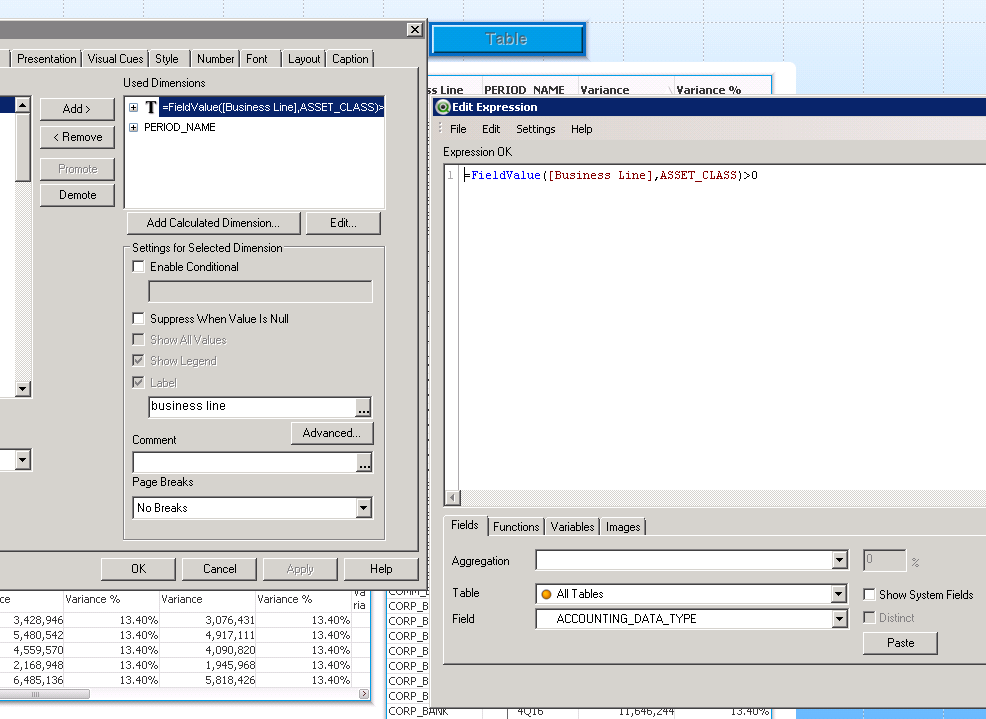# QlikView Scripting

Discussion Board for collaboration on QlikView Scripting.

Announcements

Contributor III

## Question on Dimension List

When I'm choosing dimensions, how can I use one Expressions to get all my field values, ie:

EX: I have 2 fields business line, Asset class.

Instead of choosing each field in the dimension,  can I choose an expression (1 dimension) to

list both these fields.

I'm trying to display these fields in 1 column instead of having them individually print out across 2 columns.

GC.

4 RepliesMVP

## Re: Question on Dimension List

can you clarify with an example?

Contributor III

## Re: Question on Dimension List

there must be a function, that will let me return the values of my 2 fields, using 1 dimension expression, like:

getfield(business_line, Asset_class) > 0Contributor

## Re: Question on Dimension List

yes. Look into valuelist() along with Pick.

Contributor

## Re: Question on Dimension List

well that was weird. A recent post on a unrelated question just used the exact thing I was telling you about. Here is the link to the thread and below is the example from it. http://community.qlik.com/thread/137368

=pick(

match(

Valuelist(

'\$(=field1)', '\$(=field2)', '\$(=field3)','\$(=field4))'

)

, '\$(=field1)', '\$(=field2)', '\$(=field3)','\$(=field4)'

)

, count({1<field1 = {'\$(=field1)'}>} distinct fieldx)

, count({1<field2 = {'\$(=field2)'}>} distinct fieldx)

, count({1<field3= {'\$(=field3)'}>} distinct fieldx)

, count({1<field4= {'\$(=field4)'}>} distinct fieldx)

Community Browser